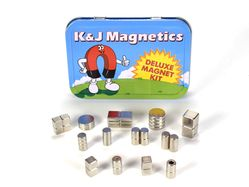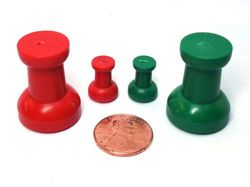Cart (0)

All orders placed & paid online by 1PM ET (M-F) will be shipped the same day!
Details

# Magnetic Units Converter

Easily convert between different magnetic units of measure

Want to read about all of these units? Click the Show All Descriptions button above!

Magnetic units can be a confusing topic. When converting from one unit to another, we often ask ourselves: "What does this unit mean?"

Below are a series of magnetic unit conversion calculators with some explanation for each. It starts with the simplest units and builds up from there.

## Magnetic FluxThe SI (metric) unit for magnetic flux is the weber (Wb). Where does this come from? What kind of magnetic strength does 1 weber represent?

Imagine encircling an area with a circle of wire. If the magnetic flux in there changes by 1 weber in a second, you'll be able to measure 1 volt induced across the wire. So: 1 weber per second equals 1 volt in the circle of wire. Or: 1 Wb = 1 V s.

Note that nothing is mentioned about the size of the loop of wire. It doesn't matter if the loop of wire is one inch or one meter across. If you're measuring 1 volt across the wire, the magnetic flux is changing by 1 weber per second in there.

=
1

## Magnetic Flux DensityThe SI unit for flux density, or induction, is the tesla (T). This property is also referred to as the B field. In the equations from our Surface Fields article, we use a "B" to denote this term.

Unlike Magnetic Flux above, the Flux Density defines some size for the loop of wire in that example. Flux Density is a measure how many webers are squeezed into some area. In fact, webers per square meter is the unit for flux density. By definition, 1 weber per square meter (Wb/m2) = 1 tesla (T).

The conversion between gauss and tesla is an easy one: 1 tesla = 10,000 gauss.

Here's a confusing part: Many people call this, "field strength." We often refer to field strength in gauss. Technically, we should probably use the term, "magnetic flux density," though it's much more commonly called, "field strength."

• We use flux density to express Surface Field.
• We use flux density to describe the "field strength" at some distance from a magnet, used in our Pull Force Calculator and in the formulas given in our Surface Fields article.
• We measure field strength with a magnetometer, expressed in gauss.
• The average flux density here on the surface of the earth is about 0.5 gauss, though it varies from 0.22 to 0.6 gauss.
• The magnetic field diagrams here at www.kjmagnetics.com use color to indicate the strength of the flux density.
=
10,000

## Field StrengthThere's another, somehow different unit for field strength. It is commonly expressed in amperes/meter (A/m) or oersted (Oe). How is this different than the flux density expressed in gauss or tesla? Why are there 2 different units? Are they the same thing?

When we think about the "field strength" at the surface of a magnet (the Surface Field), we're looking for something expressed in gauss. For example, a D82 disc magnet has a surface field of about 2,952 gauss. Stick a magnetometer on the surface of this magnet and we'll measure magnetic flux (often called field strength). So what's this oersted thing all about?

When manufacturing permanent magnets, magnet material is magnetized by exposing it to an external magnetic field. In practice, this means the magnet material is placed in a fixture, sitting inside a big coil of wire. For a brief instant, a strong magnetic field is created by running a lot of electric current through the coil of wire. The magnet is exposed to a magnetic field strong enough to magnetize the magnet. Historically and commonly, this field strength is expressed in A/m or Oe.

The demagnetization curve of a magnet material describes it magnetic properties. It describes how much field strength is required to magnetize a magnet, and more importantly for magnet users, how the magnet will perform. One axis of this curve is B (flux density expressed in gauss, comes from the magnet itself) and the other axis is H (the applied or external magnetic field in the magnetizing fixture, expressed in Oe). You can find BH curves for various magnet grades on our BH curves page. The second half of our Magnet Grades article has a great step-by-step description about how these curves are measured.

In some ways, you might argue that these things are really the same. They are in many cases, but not always. There are times when saying that 1 oersted is like 1 gauss works out well. Since B = µH, it's a fair assumption if the material is air. If you dive into the physics and math of these properties, you'll find a lot more interesting, complex stuff going on. There's a lot more to learn than we'll go into here in a simple unit converter!

=
79.5775

## BHmax - Maximum Energy ProductBHmax is a single number that describes the strength of a magnet. It is expressed in mega gauss oersteds (MGOe). It is the product of B x H on the demagnetization curve, at the location where this number works out to be the highest. In other words, it's the area shown in the box under the BH curve.

It also happens to be the "N" number in neodymium magnet grades. An N42 magnet has a maximum energy product of 42 MGOe, while an N52 magnet has a maximum energy product of 52 MGOe.

When comparing neodymium magnets, we find that the pull force relates directly to this number. If everything else is equal, a magnet with a BHmax that's 10% higher will have about a 10% higher pull force.

This number is great for comparing various neodymium magnet grades, or even between neodymium and samarium cobalt magnets. It is less useful when comparing very different magnet types, such as neodymium magnets vs. Alnico, since the Alnico demagnetization curve is so different.

=
413.8

## Magnetic Dipole Moment (m)

We're often asked what the dipole moment of a given magnet is? What does this mean? What is the dipole moment?

Moment is an engineering term used to describe a torque value. For example, if you turn a bolt with 2 ft long wrench, applying 10 lb of force on the end of the wrench, you would be turning the bolt with 10 x 2 = 20 ft lb of torqueIf you're metrically inclined, you might describe a 44.5 Newton force on a 0.61 meter wrench, yielding a 27.1 N m torque..

By definition, the magnetic dipole moment is the turning moment (the torque that's trying to rotate the magnet) that a magnet "feels" in a given magnetic field. Imagine placing a magnet inside a space that has a strong magnetic field, like the inside of an MRI machine. The magnetic dipole moment is how much torque will try rotating the magnet to align with the MRI's field.

What's a more practical, real world example? Consider a compass needle. The needle itself is a magnet. If it's not pointing north (aligning itself with the earth's magnetic field), the needle will feel a torque trying to rotate it towards north. It's a fairly weak torque, because the earth's magnetic field is so weak (about 0.5 gauss).

Units for dipole moment make sense with this mechanical description: newton meters per Tesla (N m / T). That's newton meters (the torque, force times distance) per Tesla (the field). If you're not used to metric units, you might say the same thing as pound-feet of torque per gauss (lb ft / G). Though technically correct, we've never seen anyone use this unit.

Much more commonly, this unit is expressed in Ampere square meters, A m2. Electrical engineers and physicists find this useful. It's an easy conversion: 1 A m2 = 1 N m / T. You can calculate the dipole moments value yourself using this formula dipole moment formula. Or use our online Pull Force Calculator, which calculates the dipole moment.

=
1000

## Who are all of these units named after?

Many magnetic units are named for the people who discovered or invented these ideas in our not so distant history. Learn more about them at the links below, listed in order of appearance.The volt is named after the Italian physicist and chemist Alessandro Volta (17451827), inventor of the electrical battery and discoverer of methane.The ampere is named after the French physicist and mathematician André-Marie Ampère (1775-1836). One of the founders of the science of classical electromagnetism, he referred to the subject as "electrodynamics."The gauss is named after Johann Carl Friedrich Gauss (1777-1855). Gauss was a German mathematician who contributed significantly to many, many fields. Gauss had an exceptional influence in many fields of mathematics and science and is ranked as one of history's most influential mathematicians.The oersted is named after the Danish physicist and chemist Hans Christian Ørsted (1777-1851), who discovered that electric currents create magnetic fields, an important aspect of electromagnetism. He is still known today for Oersted's Law.The weber is named after the German physicist Wilhelm Eduard Weber (18041891). Invented the first electromagnetic telegraph with Gauss.The joule is a unit of energy named after the English physicist James Prescott Joule (1818-1889). Joule studied the nature of heat, and discovered its relationship to mechanical work and energy. This led to the law of conservation of energy and the first law of thermodynamics.The maxwell is named after the Scottish scientist James Clerk Maxwell (1831-1879). His most notable achievement was to formulate the classical theory of electromagnetic radiation, bringing together electricity, magnetism, and light as manifestations of the same phenomenon. Maxwell's equations for electromagnetism have been called the "second great unification in physics."The tesla is named after Nikola Tesla (1856-1943). The Serbian American inventor, electrical engineer, mechanical engineer, physicist, and futurist is best known for his contributions to the design of the modern alternating current (AC) electricity supply system.

Would likely have enjoyed driving this all-electric car.

This page provides easy conversions for a number of magnetic units. If you find any errors, be sure to let us know!

November
Specials10% Off
TIN1
Deluxe magnet kit, great gift idea!10% Off

Holiday magnetic thumbtack kits10% Off
WPH-LG
Waterproof hook magnet, great for hanging decor

Order online for
fastest shipping!

No Minimum Order!

K&J Magnetics, Inc.
Your online source for incredibly powerful neodymium magnets